Courses

# NCERT Solutions(Part- 1)- Understanding Quadrilaterals Class 8 Notes | EduRev

## Mathematics (Maths) Class 8

Created by: Vp Classes

## Class 8 : NCERT Solutions(Part- 1)- Understanding Quadrilaterals Class 8 Notes | EduRev

The document NCERT Solutions(Part- 1)- Understanding Quadrilaterals Class 8 Notes | EduRev is a part of the Class 8 Course Mathematics (Maths) Class 8.
All you need of Class 8 at this link: Class 8

EXERCISE 3.1
Question 1. Given here are some figures.
1.2.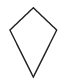3.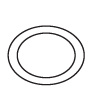4.5.6.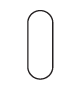7.8.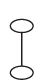Classify each of them on the basis of the following:
(a) Simple curve

(b) Simple closed curve
(c) Polygon
(d) Convex polygon

(e) Concave polygon
Solution:
Simple curve: A simple curve is a curve that does not cross itself.
Simple closed curve:
In simple closed curves the shapes are closed by line-segments or by a curved lines.
Polygon:
A simple closed curve made up of only line segments is called a polygon.

Convex polygon: A Convex polygon is defined as a polygon with no portions of their diagonals in their exteriors.
Concave Polygon: A concave polygon is defined as a polygon with one or more interior angles greater than 180°.
(a) Simple curves are: (1), (2), (5), (6) and (7).
(b) Simple closed curves are: (1), (2), (5), (6) and (7).
(c) Polygons are: (1), (2)
Note: '4' is not a polygon because polygon is a simple closed curve made up of
line segments and the 4th figure is not a simple curve because it crosses itself. Therefore, '4' is not a polygon.
In the latest edition, this figure is no longer listed as the polygon.
(d) Convex polygon is: (2)
(e) Convex polygons are (1)

Question 2. How many diagonals does each of the following have?
(b) A regular hexagon
(c) A triangle
Solution:
A diagonal is a line segment connecting two non-consecutive vertices of a polygon. Draw the above given polygon and mark vertices and then, draw lines joining the two non-consecutive vertices. From this, we can calculate the number of diagonals.
A convex quadrilateral has two diagonals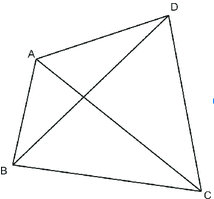Here, AC and BD are two diagonals.
(b) Regular hexagon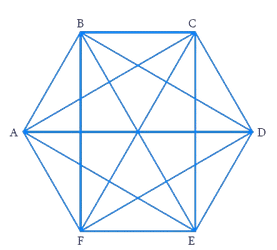Here, the diagonals are AD, AE, BD, BE, FC, FB, AC, EC and FD. Totally there are 9 diagonals.
(c) A triangle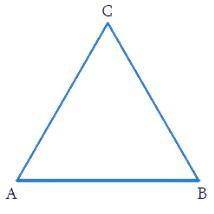A triangle has no diagonal because there no two non-consecutive vertices.
Note: Number of diagonals in a polygon of n-sides =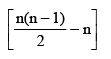Question 3. What is the sum of the measures of the angles of a convex quadrilateral? Will this property hold if the quadrilateral is not convex? (Make a non-convex quadrilateral and try!)
Solution: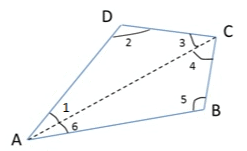ABCD is a convex quadrilateral made of two triangles ΔABC and ΔADC. We know that the sum of the angles of a triangle is 180 degree. So,
∠6+∠5+∠4 = 180° (Sum of the angles of ΔABC is 180°)
∠1+∠2+∠3 = 180° (Sum of the angles of ΔADC is 180°)
Adding the above equations, we get
∠6+∠5+∠4+∠1+∠2+∠3 = 360°
On Rearranging the terms,
∠6+∠1+∠3+∠4+∠5+∠2 = 360°
∠A+∠C+∠B+∠D = 360° (∠6+∠1 = ∠A, ∠3+∠4 = ∠C  )
Hence, the sum of measures of the triangles of a convex quadrilateral is 360.

Yes, even if quadrilateral is not convex then, this property applies. Let ABCD be a non-convex quadrilateral; join BD, which also divides the quadrilateral in two triangles.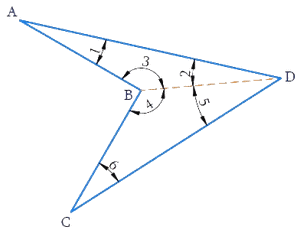Using the angle sum property of triangle again, ABCD is a concave quadrilateral, made of two triangles ΔABD and ΔBCD. Therefore, the sum of all the interior angles of this quadrilateral will also be,
180+180 = 360

Question 4. Examine the table. (Each figure is divided into triangles and the sum of the angles deduced from that).

 Figure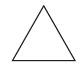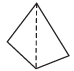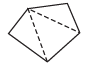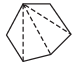Side 3 4 5 6 Angle sum 180° 2 x 180°= (4 – 2) x 180° 3 x 180°= (5 – 2) x 180° 4 x 180°= (6 – 2) x 180°

What can you say about the angle sum of a convex polygon with number of sides? (a) 7 (b) 8 (c) 10 (d) n
Solution:

From the above table, we conclude that sum of the interior angles of polygon with n-sides = (n – 2) x 180°
(a) When n = 7

Substituting n = 7 in the above formula, we have
Sum of interior angles of a polygon of 7 sides (i.e. when n = 7)
= (n – 2) x 180° = (7 – 2) x 180°
= 5 x 180° = 900°

(b) When n = 8
Substituting n = 8 in the above formula, we have
Sum of interior angles of a polygon having 8 sides
= (n – 2) x 180° = (8 – 2) x 180°
= 6 x 180° = 1080°

(c) When n = 10
Substituting n = 10 in the above formula, we have

Sum of interior angles of a polygon having 10 sides
= (n – 2) x 180°
= (10 – 2) x 180°
= 8 x 180° = 1440°

(d) When n = n
The sum of interior angles of a polygon having n-sides = (n – 2) x 180°

Question 5. What is a regular polygon?
State the name of a regular polygon of following sides:
(i) 3 sides
(ii) 4 sides
(iii) 6 sides

Solution: A polygon is said to be a regular polygon if
(a) The measures of its interior angles are equal and
(b) The lengths of its sides are equal
(i) A regular polygon of three sides is called an equilateral Triangle.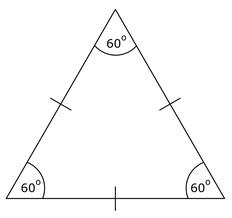(ii) A regular polygon of 4 sides is called square.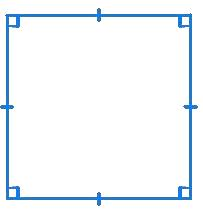(iii) A regular polygon of 6 sides is called regular hexagon.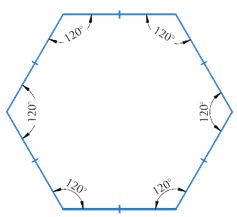Question 6. Find the angle measure x in the following figures.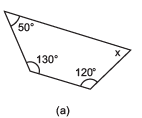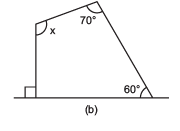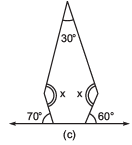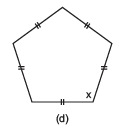Solution:
(a) The figure is having four sides. Hence, it is a quadrilateral.
As, Sum of interior angles of a quadrilateral = 360°
x + 120° + 130° + 50° = 360°
⇒ x + 300° = 360°
x = 360° – 300° = 60°

(b) The figure is having four sides. Hence, it is a quadrilateral. Also, one side is perpendicular.
As, sum of interior angles of a quadrilateral = 360°
The figure is having four sides. Hence, it is a quadrilateral.

x + 60° + 70° + 90° = 360°
x + 220° = 360°
x = 360° – 220° = 140°

(c) The figure is having 5 sides.Hence, it is a pentagon.
Sum of interior angles of a pentagon = 540°
Two angles at the bottom are forming linear pair.
∴ 180° - 70° = 110°
180° – 60° = 120°
Interior angles are: 30°, x°, x°, 110° and 120°
30° + x + x + 110° + 120° = 540°
2x + 260° = 540°
2x = 280°
x = 140°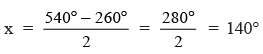(d) The figure is having 5 equal sides. Hence, It is a regular pentagon. Thus, its al angles are equal.
Sum of all interior angles of a regular pentagon = 540°.

x + x + x + x + x = 540°
5x = 540°
x = 540° ÷ 5 = 108°

Question 7.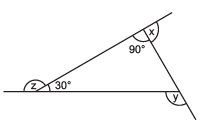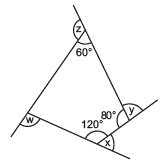(a) Find x + y + z.
(b) Find x + y + z + w.
Solution:
(a) Sum of all the angles of a triangle = 180°

∴ One angle of a triangle is 180° - (90°+30°) = 60°
x + 90° = 180° (Linear pair)
⇒ x = 180° – 90° = 90°
y = 30° + 90° = 120° (∵ Sum of interior opposite angles = exterior angle)

z = 180° – 30° = 150° (Linear pair)
Now, x + y + z = 90° + 120° + 150° = 360°

(b) Sum of interior angles of a quadrilateral = 360°
∴ ∠1 + 120° + 80° + 60° = 360°
∠1 + 260° = 360°
1 = 360° – 260° = 100°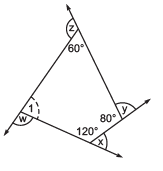Now, x + 120° = 180° (Linear pair)
∴ x = 180° – 120° = 60°
y + 80° = 180°   (Linear pair)
∴  y = 180° – 80° = 100°
z + 60 = 180° (Linear pair)
∴  z = 180° – 60° = 120°
w + 100 = 180°  (Linear pair)
∴ w = 180° – 100° = 80°
Thus, x + y + z + w = 60° + 100° + 120° + 80° = 360°

Offer running on EduRev: Apply code STAYHOME200 to get INR 200 off on our premium plan EduRev Infinity!

,

,

,

,

,

,

,

,

,

,

,

,

,

,

,

,

,

,

,

,

,

;